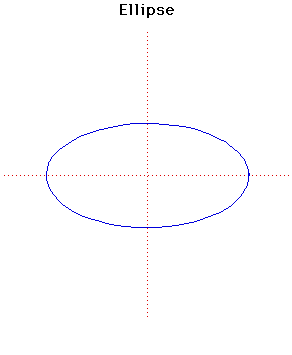# Curves

### EllipseCartesian equation:
$x^{2}/a^{2} + y^{2}/b^{2} = 1$
or parametrically:
$x = a \cos(t), y = b \sin(t)$

### Description

The ellipse was first studied by Menaechmus. Euclid wrote about the ellipse and it was given its present name by Apollonius. The focus and directrix of an ellipse were considered by Pappus.

Kepler, in 1602, said he believed that the orbit of Mars was oval, then he later discovered that it was an ellipse with the sun at one focus. In fact Kepler introduced the word "focus" and published his discovery in 1609. The eccentricity of the planetary orbits is small (i.e. they are close to circles). The eccentricity of Mars is 1 /11 and of the Earth is 1 /60 .

In 1705 Halley showed that the comet, which is now called after him, moved in an elliptical orbit round the sun. The eccentricity of Halley's comet is 0.9675 so it is close to a parabola (eccentricity 1).

The area of the ellipse is $\pi ab$. There is no exact formula for the length of an ellipse in elementary functions and this led to the study of elliptic functions. Ramanujan, in 1914, gave the approximate length
$\pi(3(a + b) - √[(a + 3b)(3a + b)])$.
The pedal curve of an ellipse, with its focus as pedal point, is a circle.

The evolute of the ellipse with equation given above is the Lamé curve.
$(ax)^{2/3} + (by)^{2/3} = (a - b)^{2/3}$.
From a point inside the evolute (it is a closed curve) four normals can be drawn to the ellipse but from a point outside only two normals can be drawn.

Other Web site:

Xah Lee

### Associated Curves

Definitions of the Associated curves